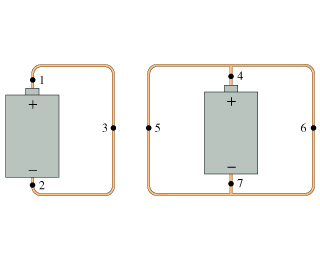# Problem: The two circuits in (Figure 1) use identical batteries and wires of equal diameters. Rank in order, from largest to smallest, the currents I1 to I7 at points 1 to 7.

###### FREE Expert Solution

We can solve this problem using Ohm's law and the law of conservation of charge.

Ohm's law:

$\overline{){\mathbit{V}}{\mathbf{=}}{\mathbit{I}}{\mathbit{R}}}$

In the first circuit, the current is flowing towards point 1.

The potential difference across the points 1, 2, and 3 are the same.

From Ohm's law, current, I = V/R.

Thus, from the conservation of charge, currents at points 1, 2, and 3 are equal.

I= I2 = I3 = ΔV/R

99% (27 ratings)###### Problem Details

The two circuits in (Figure 1) use identical batteries and wires of equal diameters. Rank in order, from largest to smallest, the currents I1 to I7 at points 1 to 7.